MATH 1280 Introduction to Statistics – Discussion Unit 4 – Part 1

Sandro Botticelli’s painting

Random variables are used to model situations in which the outcome, before the fact, is uncertain. One component in the model is the sample space.”

The sample space is the list of all possible outcomes (or a range of possible values). For each value in the sample space, there is an associated probability.”

The probability can either be an estimate of something that exists in the real world or it could be an exact value that comes from an ideal distribution.”

Sometimes identifying the sample space helps to remind you exactly what it is that you are measuring.”

Think of something that you might want to measure that is affected by random variation.”

Identify what you want to measure, then describe its (approximate) sample space.”

Give a rough description of the probabilities associated with those values (you can simply specify if they are all the same probability or if values in one range will be more likely than values in another range).”

Painted by Sandro Botticelli

What would you say to a person who says that he or she "knows" what the outcome of an individual observation will be (an outcome of something that has not happened yet that is subject to random error)?”

When forming your answer to this question you may give an example of a situation from your own field of interest for which a random variable can serve as a model.”

One of great paintings by Sandro Botticelli

There is no requirement to use sources from the Internet, but if you use an idea or a quotation from any source, it should be cited (such as putting the author and year at the end of the sentence and then adding a reference at the end to describe the source).”

# Please find the answer on following post: MATH 1280 Introduction to Statistics – Discussion Unit 4 – Part 2

1.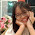This content I've tried to read till the end, is being blanked me too much headache

1.Thank you to visit:
MATH 1280 Introduction to Statistics – Discussion Unit 4 – Part 1
-
Sandro Botticelli’s painting

2.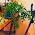Beautiful Boticelli.

1.Thank you to visit:
MATH 1280 Introduction to Statistics – Discussion Unit 4 – Part 1
-
“Random variables are used to model situations in which the outcome, before the fact, is uncertain. One component in the model is the sample space.”

3.Beautiful pictures of Boticelli.

1.Thank you to visit:
MATH 1280 Introduction to Statistics – Discussion Unit 4 – Part 1
-
“The sample space is the list of all possible outcomes (or a range of possible values). For each value in the sample space, there is an associated probability.”

4.Thanks for your sharing 😊

1.Thank you to visit:
MATH 1280 Introduction to Statistics – Discussion Unit 4 – Part 1
-
“The probability can either be an estimate of something that exists in the real world or it could be an exact value that comes from an ideal distribution.”

5.This is interesting like always
xx

1.Thank you to visit:
MATH 1280 Introduction to Statistics – Discussion Unit 4 – Part 1
-
“Sometimes identifying the sample space helps to remind you exactly what it is that you are measuring.”

6.Those paintings are beautiful☺

1.Thank you to visit:
MATH 1280 Introduction to Statistics – Discussion Unit 4 – Part 1
-
“Think of something that you might want to measure that is affected by random variation.”

7.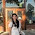Very nice paintings!! Kisses

1.Thank you to visit:
MATH 1280 Introduction to Statistics – Discussion Unit 4 – Part 1
-
“Identify what you want to measure, then describe its (approximate) sample space.”

8.Ayy I took statistics in school and wasn't very good at it. LOL!

1.Thank you to visit:
MATH 1280 Introduction to Statistics – Discussion Unit 4 – Part 1
-
“Give a rough description of the probabilities associated with those values (you can simply specify if they are all the same probability or if values in one range will be more likely than values in another range).”

9.Thanks for sharing. :-)

Best regards

1.Thank you to visit:
MATH 1280 Introduction to Statistics – Discussion Unit 4 – Part 1
-
Painted by Sandro Botticelli

10.Piękne obrazy. Co do treści nie wiem czy dobrze zrozumiałam, ale wydaje mi się, że tu chodzi o to, iż nigdy do końca nie wiadomo co będzie.? :)

1.Thank you to visit:
MATH 1280 Introduction to Statistics – Discussion Unit 4 – Part 1
-
“What would you say to a person who says that he or she "knows" what the outcome of an individual observation will be (an outcome of something that has not happened yet that is subject to random error)?”

11.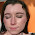Looks like a good task.

1.Thank you to visit:
MATH 1280 Introduction to Statistics – Discussion Unit 4 – Part 1
-
“When forming your answer to this question you may give an example of a situation from your own field of interest for which a random variable can serve as a model.”

12.Stunning paintings by Botticelli - and they are so large when you see them in real life.

1.Thank you to visit:
MATH 1280 Introduction to Statistics – Discussion Unit 4 – Part 1
-
One of great paintings by Sandro Botticelli

13.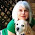I am lost in statistics but I enjoyed Sandro Botticelli paintings.
Wish you a nice and peaceful week!

1.I see....

Thank you to visit:
MATH 1280 Introduction to Statistics – Discussion Unit 4 – Part 1
-
“There is no requirement to use sources from the Internet, but if you use an idea or a quotation from any source, it should be cited (such as putting the author and year at the end of the sentence and then adding a reference at the end to describe the source).”

14.wow so stunning paintings:)

1.Thank you to visit:
MATH 1280 Introduction to Statistics – Discussion Unit 4 – Part 1

# Please find the answer on following post: MATH 1280 Introduction to Statistics – Discussion Unit 4 – Part 2

15.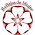Have a nice week

1.Thank you to visit:
MATH 1280 Introduction to Statistics – Discussion Unit 4 – Part 1
-
Sandro Botticelli’s painting

16.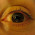Nice and informative as always! :)
--
O diário da Inês | Facebook | Instagram

17.hank you to visit:
MATH 1280 Introduction to Statistics – Discussion Unit 4 – Part 1
-